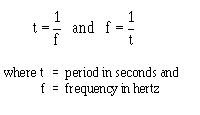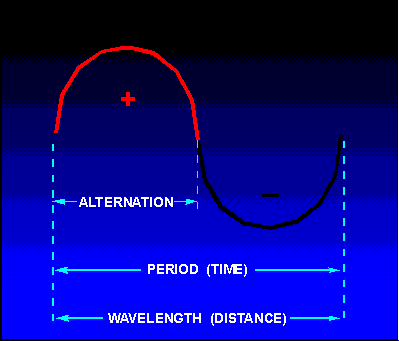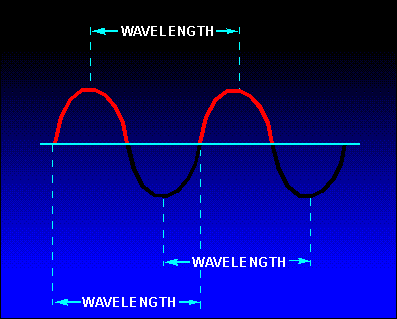Frequency alternating current or voltage completed each second is referred to as the FREQUENCY. Frequency is always measured and expressed in hertz. ">Custom SearchFREQUENCY If the loop in the figure 1-8 (A) makes one complete revolution each second, the generator produces one complete cycle of ac during each second (1 Hz). Increasing the number of revolutions to two per second will produce two complete cycles of ac per second (2 Hz). The number of complete cycles of alternating current or voltage completed each second is referred to as the FREQUENCY. Frequency is always measured and expressed in hertz. Alternating-current frequency is an important term to understand since most ac electrical equipments require a specific frequency for proper operation. Q.20 Define Frequency. PERIOD An individual cycle of any sine wave represents a definite amount of TIME. Notice that figure 1-10 shows 2 cycles of a sine wave which has a frequency of 2 hertz (Hz). Since 2 cycles occur each second, 1 cycle must require one-half second of time. The time required to complete one cycle of a waveform is called the PERIOD of the wave. In figure 1 - 10, the period is one-half second. The relationship between time (t) and frequency (f) is indicated by the formulasFigure 1-10. - Period of a sine wave.Each cycle of the sine wave shown in figure 1-10consists of two identically shaped variations in voltage. The variation which occurs during the time the voltage is positive is called the POSITIVE ALTERNATION. The variation which occurs during the time the voltage is negative is called the NEGATIVE ALTERNATION. In a sine wave, these two alternations are identical in size and shape, but opposite in polarity. The distance from zero to the maximum value of each alternation is called the AMPLITUDE. The amplitude of the positive alternation and the amplitude of the negative alternation are the same. WAVELENGTH The time it takes for a sine wave to complete one cycle is defined as the period of the waveform. The distance traveled by the sine wave during this period is referred to as WAVELENGTH. Wavelength, indicated by the symbol λ (Greek lambda), is the distance along the waveform from one point to the same point on the next cycle. You can observe this relationship by examining figure 1-11. The point on the waveform that measurement of wavelength begins is not important as long as the distance is measured to the same point on the next cycle (see figure 1-12). Figure 1-11. - Wavelength.Figure 1-12. - Wavelength measurement.Q.21 What term is used to indicate the time of one complete cycle of a waveform? Q.22 What is a positive alternation? Q.23 What do the period and the wavelength of a sine wave measure, respectively?Integrated Publishing, Inc. - A (SDVOSB) Service Disabled Veteran Owned Small Business